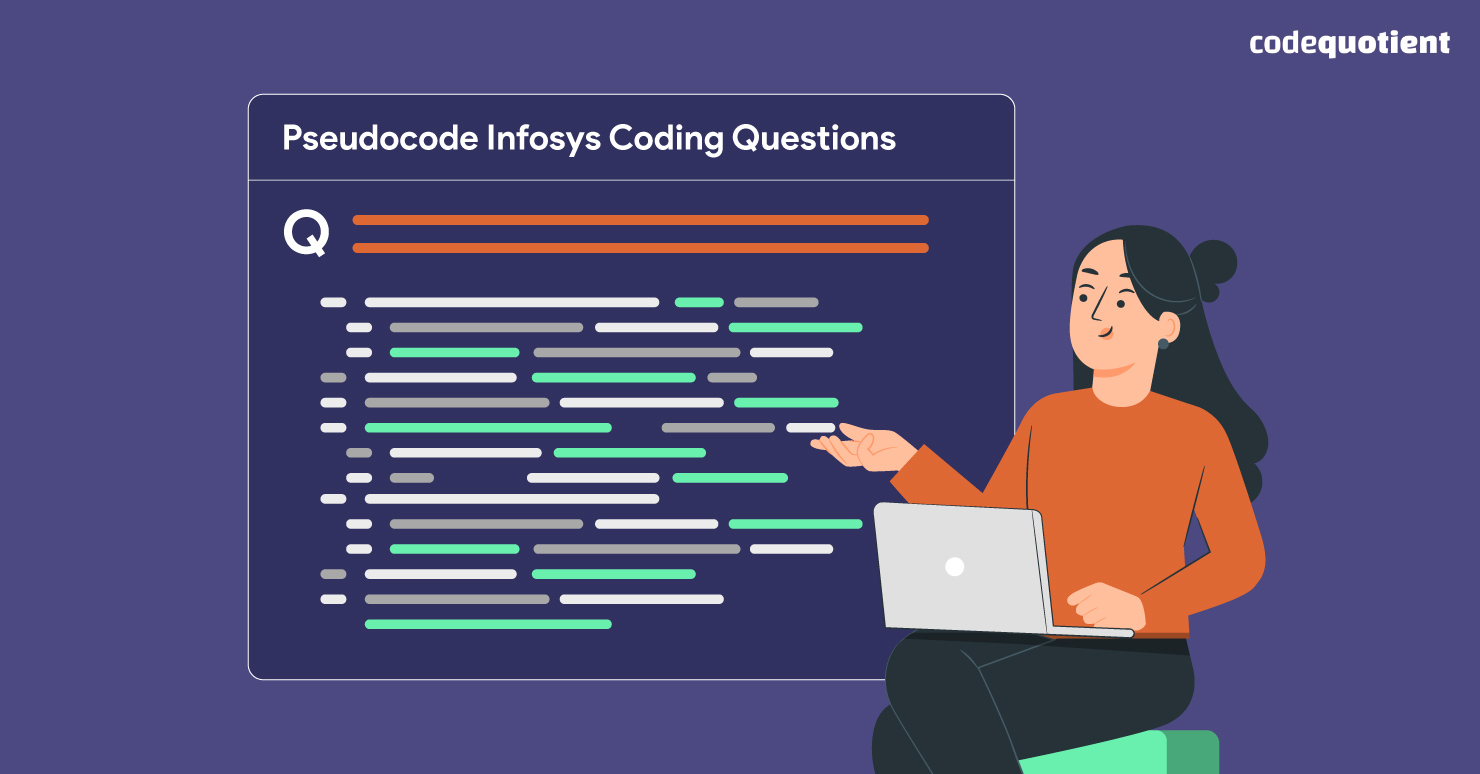In today’s world of technology, having great coding skills has become increasingly important for landing good software development jobs. Companies like Infosys, a global IT consulting and services firm, often use coding questions to assess candidates’ problem-solving skills during their hiring process. One key aspect of Infosys’ coding assessments is the Pseudocode round, which tests candidates’ ability to write algorithmic solutions using simplified code.

## What is Pseudocode?

Pseudocode is a high-level description of a computer program or algorithm that uses natural language or simplified syntax to express the logic of the program. It is not a programming language with a specific syntax but rather a way of writing down the steps of an algorithm in a language-agnostic way that can be understood easily.

Pseudocode is often used in the early stages of software development to help programmers plan and design their programs before writing actual code. It allows developers to focus on the logic and flow of the program without getting bogged down in the details of a particular programming language.

Pseudocode typically uses simple English words and phrases and basic programming constructs such as loops, conditionals, and variables. Here’s an example of a simple pseudocode algorithm that calculates the sum of two numbers: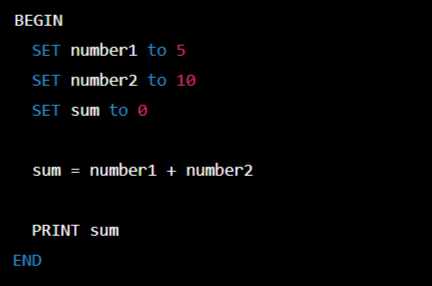## Pseudocode Infosys Coding Questions Pattern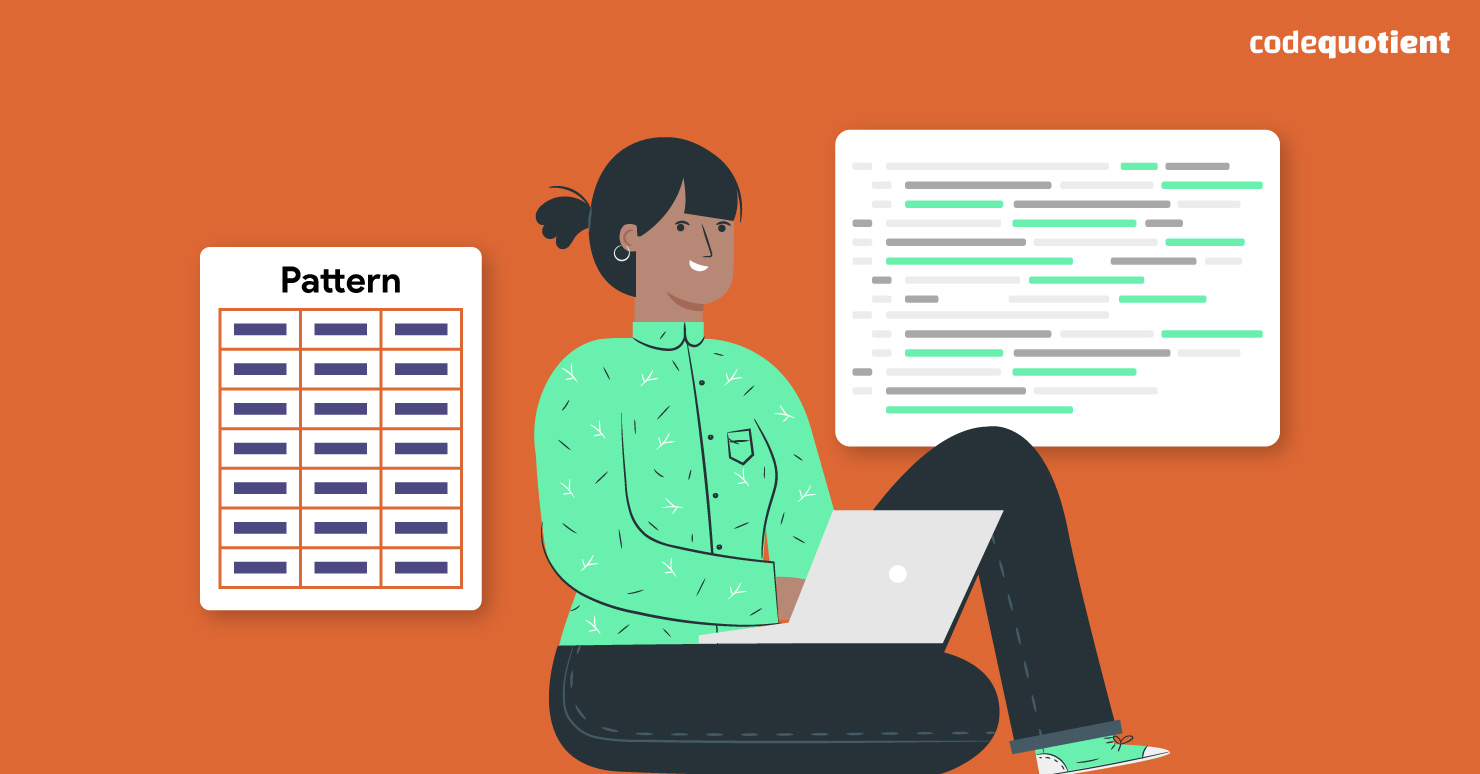The Infosys online exam, conducted annually, includes a crucial Pseudocode round. To advance to the next round, Puzzle Solving, candidates must successfully clear this round.

The Pseudocode round consists of five questions, which must be solved within a 10-minute time period. The questions are based on C, C++, and data structures, and their difficulty level ranges from moderate to high.

Also Read:  All About the CoCubes Assessment Test 2023 and Which Companies Use It

To crack the Infosys online test, performing well in this section is essential. The Pseudocode questions play a significant role in determining the candidate’s eligibility for the next round. However, there is no negative marking for this section.

Out of the five questions, one is based on the C language, one is based on the C++ language, and two are based on data structures. The questions in this section have a moderate difficulty level, but candidates can easily crack this section with practice.

## Pseudocode Infosys Coding Questions With Answers

Here are the solutions and explanations to the seven Infosys coding questions with pseudocode for 2023:

1. What could be the output of the given code?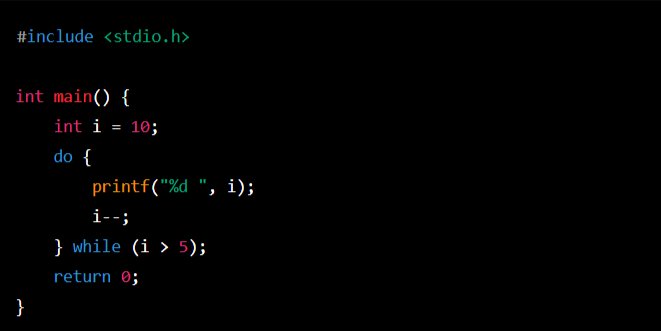Options:

1. a) 0 1 2 3 4
2. b) 1 2 3 4 5
3. c) 0 2 4 6 8
4. d) Compilation error

Answer: The correct option is a) 0 1 2 3 4. This is because the code defines a ‘while’ loop that runs as long as i is less than 5. Inside the loop, it prints the current value of iand then increments it by 1. The loop will run a total of 5 times, with ’i’ taking the values 0, 1, 2, 3, and 4. Therefore, the program’s output will be “0 1 2 3 4“.

The output: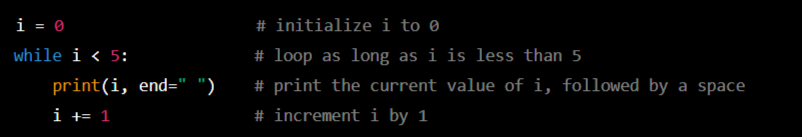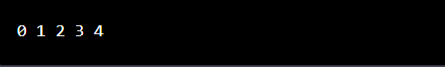1. What is the possible output of the given code?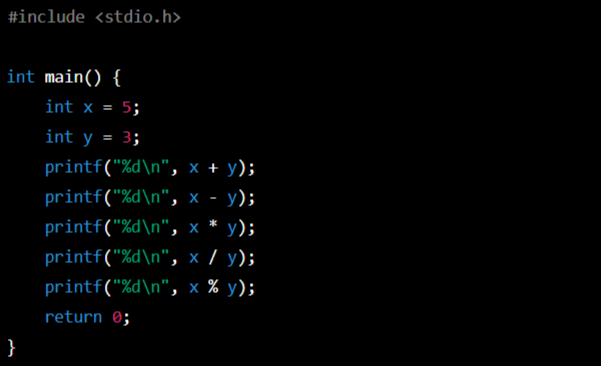Options:

1. a) 8 2 15 1 2
2. b) 8 2 15 1 1
3. c) 8 2 15 2 2
4. d) Compilation error

Answer: The correct option is b) 8 2 15 1 1. This is because the code defines two integer variables, ‘x’ and ‘y’, with values ‘ 5’ and ‘ 3’, respectively. It then prints the results of several arithmetic operations involving x and y, using the format string %d to indicate that integers should be printed. The operations are addition, subtraction, multiplication, division, and modulo. The expected output can be calculated as follows: ‘5 + 3 = 8’, ‘5 – 3 = 2’,’ 5 * 3 = 15’, ‘5 / 3 = 1’ (integer division), and 5 % 3 = 2 (remainder when 5 is divided by 3). Therefore, the output of the program will be “8\n2\n15\n1\n1\n“.

Also Read:  A Guide to Cognizant Aptitude Questions and Exam Pattern for GenC 2023

The output: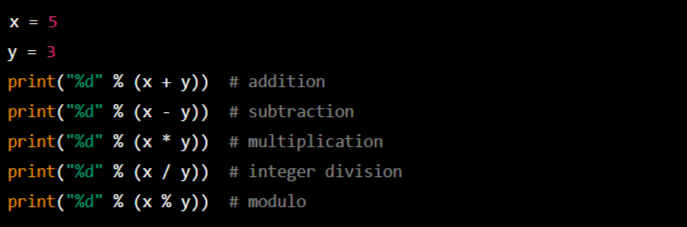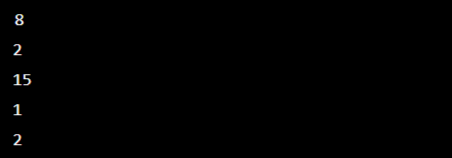1. What will be the outcome of the following code?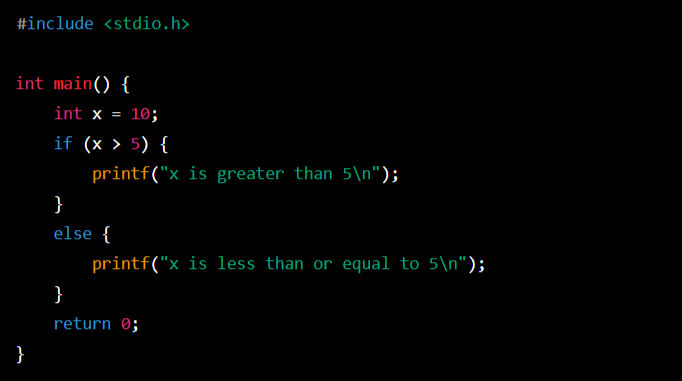Options:

1. a) x is greater than 5
2. b) x is less than or equal to 5
3. c) x is greater than or equal to 5
4. d) Compilation error

Answer: The correct option is a) x is greater than 5. This is because the code defines an integer variable ‘x’ with value 10 and then checks whether xis greater than 5 using an if’ statement. If ‘ x’ is greater than 5, the program prints the string “x is greater than 5\n”. Otherwise, it prints the string “x is less than or equal to 5\n”. Since x is indeed greater than 5, the program’s output will be “x is greater than 5\n.

The output: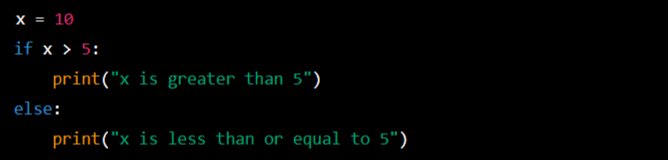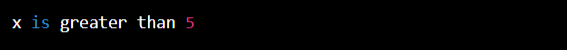1. Give the output of the following program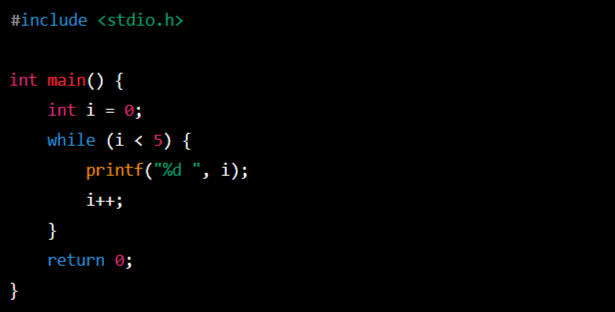Options:

1. a) 10 9 8 7 6
2. b) 9 8 7 6 5
3. c) 10 8 6
4. d) Compilation error

Answer: The correct option is a) 10 9 8 7 6. This is because the code defines an integer variable “i’’ with an initial value 10 and then enters a “do-while” loop that runs as long as iis greater than 5. Inside the loop, it prints the value of ‘i’ using the format string “%d” and then decrements “i” by 1 using the postfix decrement operator “–”. Since i is initially 10, the first value printed will be 10, followed by 9, 8, 7, and 6. Therefore, the program’s output will be “10 9 8 7 6“.

The output: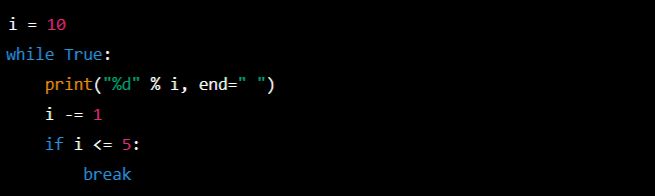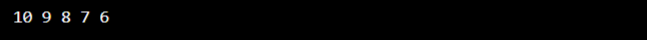1. What will be the output of the given code?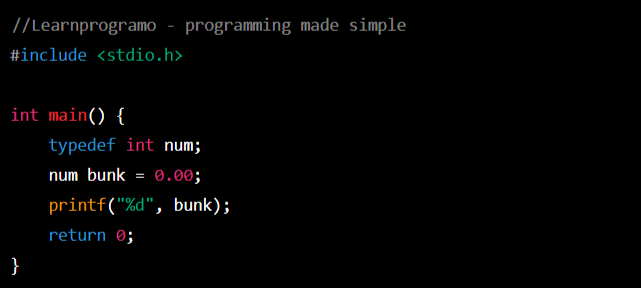Options:

1. a) 0.0
2. b) 0
3. c) logical Error
4. d) None

Answer: The program defines a new type, “num”, using “typedef”, which is equivalent to the built-in “int” type. It then declares a variable “bunk” of type “num” and initialises it to “0.00”, which is a floating-point constant. Finally, it prints the value of “bunk” using the “%d” format specifier intended for printing integers.

Since “bunk” is declared as an “int”, the floating-point constant “0.00” will be implicitly converted to an integer value of “0”. Therefore, the output of the program will be “0”.

The output: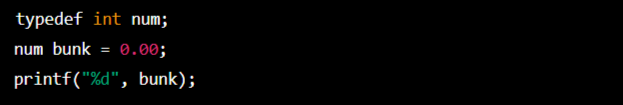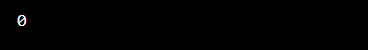## Summing up

Regularly practising Infosys coding questions and utilising Pseudocode to tackle challenging problems can enhance your problem-solving abilities, which increases your chances of passing the Infosys coding round. It’s crucial to remember that coding is a skill that necessitates perseverance and practice to excel.

If you require assistance, you can avail of CodeQuotient’s exclusive SuperCoders Program, which provides hands-on practice with full-stack development and paid internships. The program’s unique emphasis on internships helps learners develop a strong fundamental understanding that will remain with them for a long time. Apply today to this exclusive program.

## Get UGC Approved BCA DegreeAnd Earn While You Do That

Join CodeQuotient's Software Engineering Program

Study at NAAC A+ Accredited Partner University